# Lecture 30: Network Flow III

$\def\opt{ {\mathrm{opt}} } \def\val{ {\mathrm{val}} } \def\capacity{ {\mathrm{cap}} }$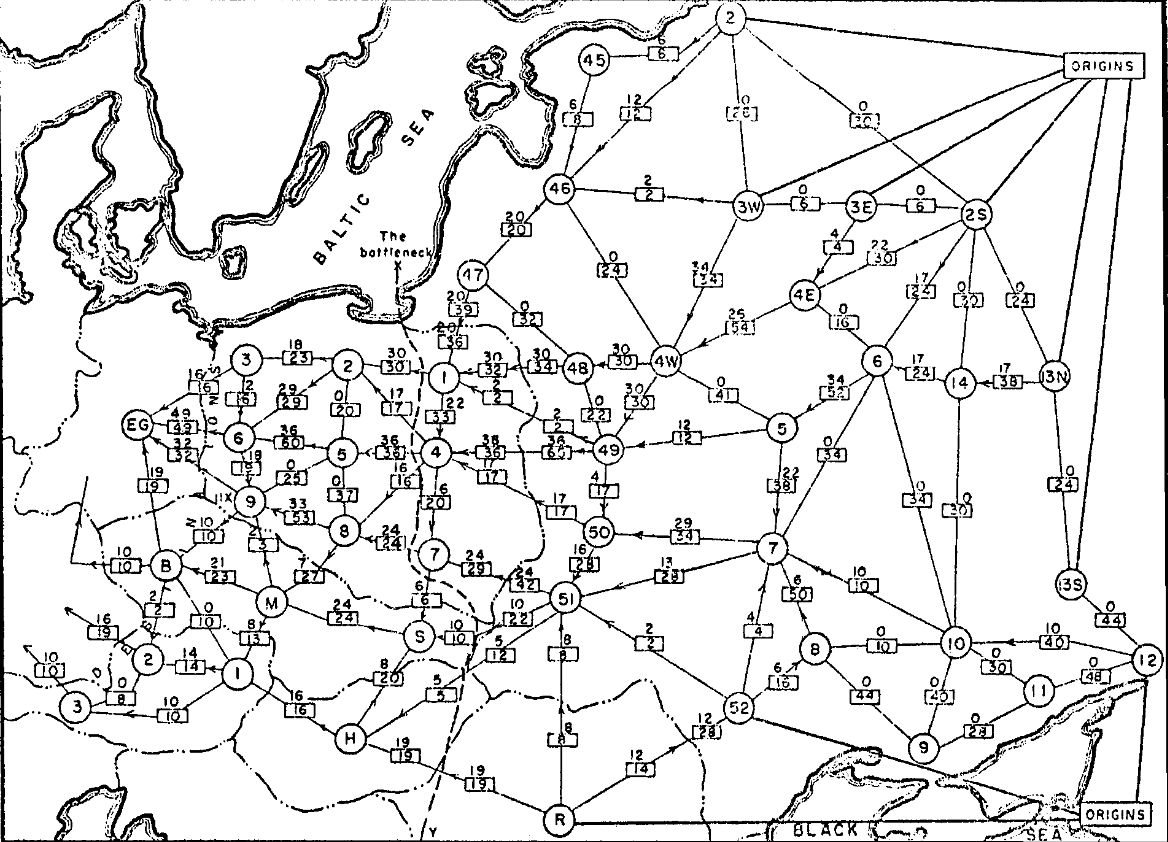## Annoucement

Midterm II on Wednesday

• Practice solutions coming soon

## Last Time

Max Flow Problem:

Input.

• weighted directed graph $G = (V, E)$
• weights = edge capacities $> 0$
• source $s$, sink $t$
• all edges oriented out of $s$
• all edges oriented into $t$

Output.

• flow $f$ of maximum value
• $\val(f) = \sum_{s \to v} f(s, v)$

## We Showed

Greedy strategy doesn’t always work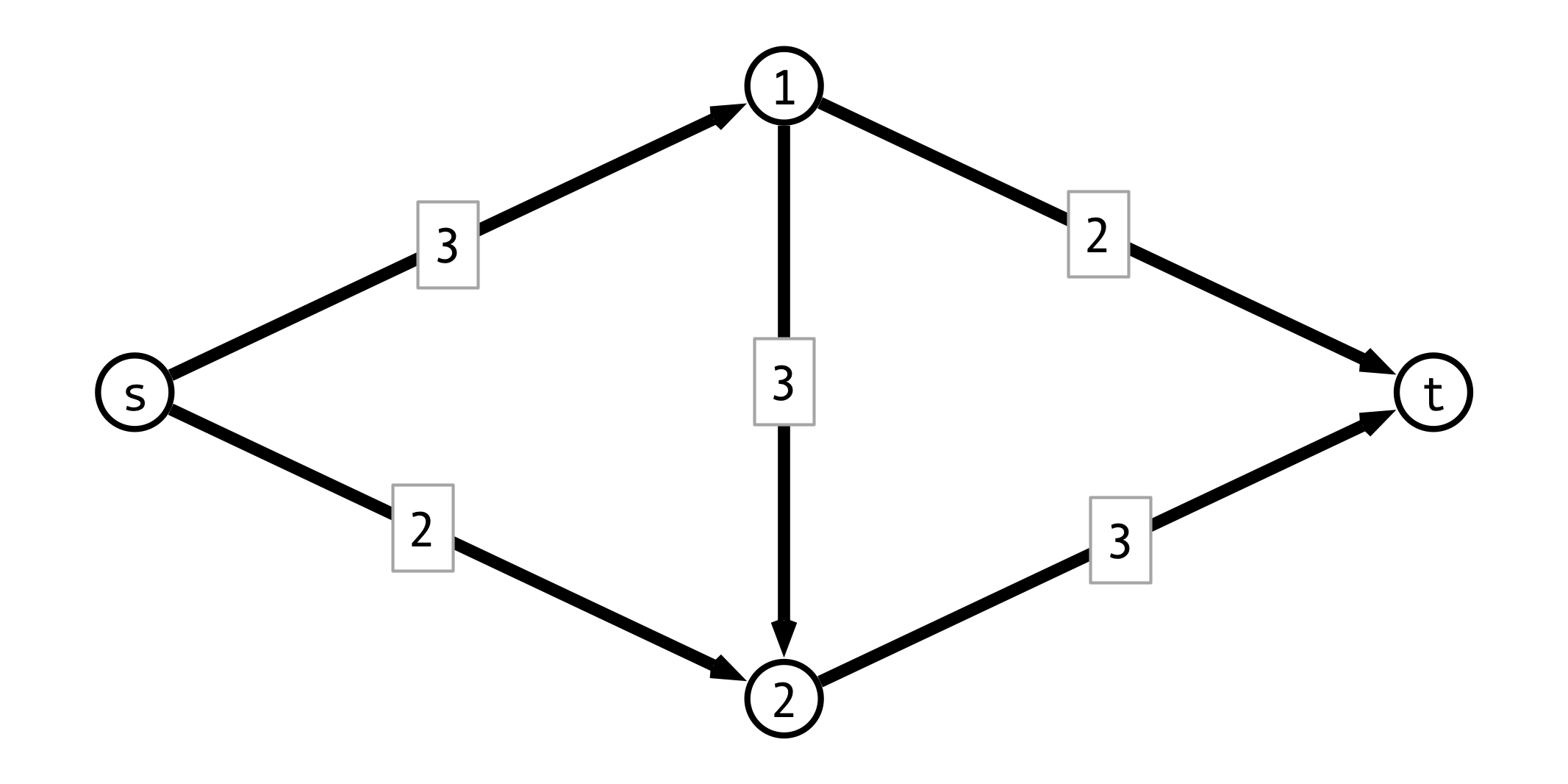## Ford-Fulkerson Idea

Given a flow $f$:

• allow forward flow to be “undone”
• when routing forward flow $f(u, v)$ across edge $(u, v)$, create backwards edge $(v, u)$ with capacity $f(u, v)$
• graph with backwards edges = residual graph
• backwards flow cancels out forward flow

Ford-Fulkerson Algorithm:

1. apply greedy strategy to residual graph
2. update residual graph with new flow
3. continue until no unsaturated path from $s$ to $t$ remains

## Questions

How do we…

1. find augmenting path $P$ from $s$ to $t$?

2. update flow $f$ according to $P$?

3. update residual graph $G_f$?

## Formalizing Ford-Fulkerson

  MaxFlow(G, s, t):
Gf <- G
f <- zero flow
P <- FindPath(Gf, s, t)
while P is not null do:
b <- min capacity of any edge in P
Augment(Gf, f, P, b)
P <- FindPath(Gf, s, t)
endwhile
return f


## Augment Procedure

  Augment(Gf, f, P, b):
for each edge (u, v) in P
if (u, v) is forward edge then
f(u, v) <- f(u, v) + b
c(u, v) <- c(u, v) - b
c(v, u) <- c(v, u) + b
else
f(v, u) <- f(v, u) - b
c(v, u) <- c(v, u) + b
c(u, v) <- c(u, v) - b


## Running Time

Assume:

1. all capacities are integers
2. $C =$ sum of capacites of edges out of $s$

Observe:

1. How long to find augmenting path $P$?

2. How long to run Augment?

3. How many iteraions of find/augment?

Conclude: Overall running time?

## Optimality of Flow?

Question. How do we know this flow is optimal?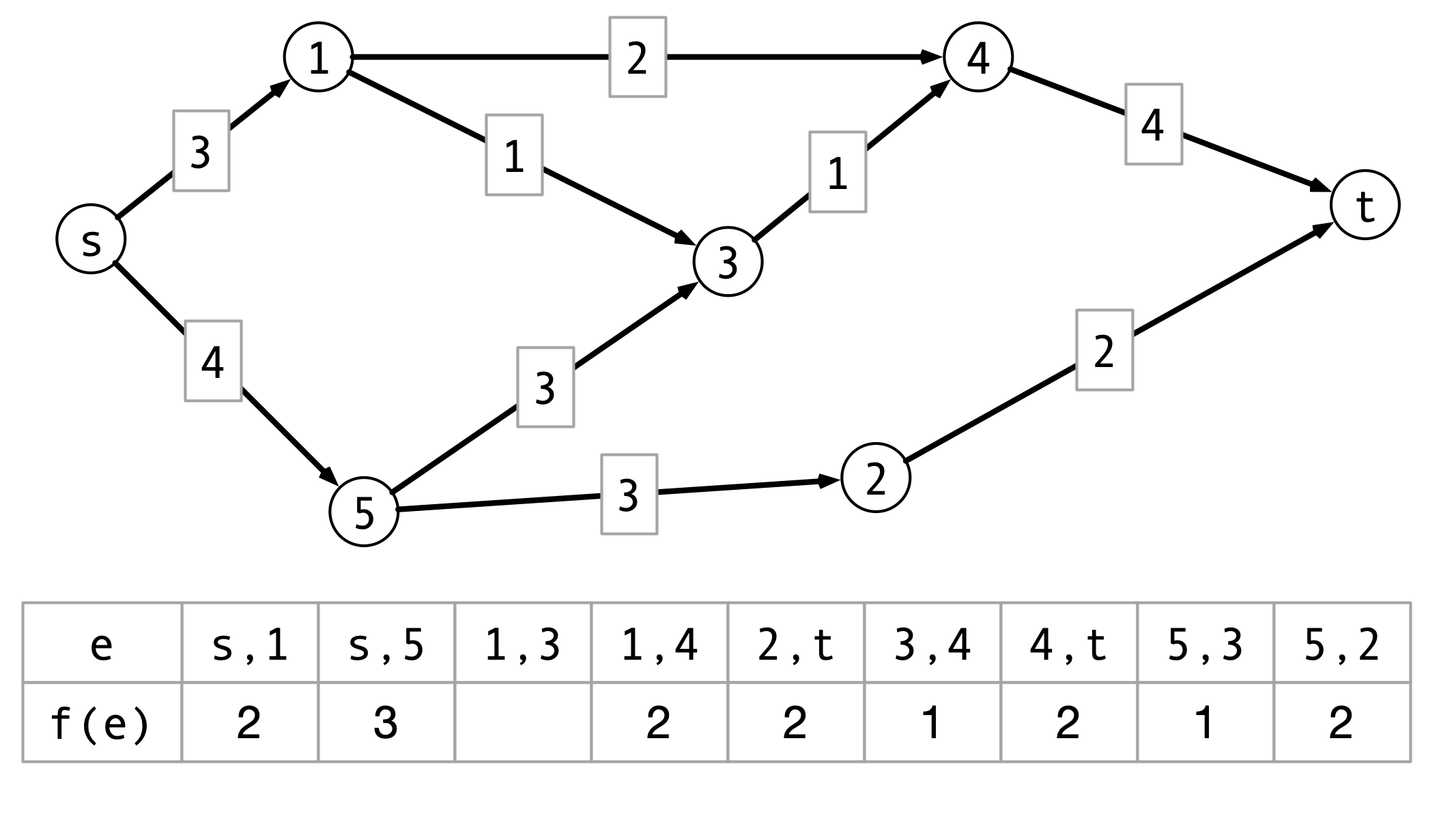## Cuts

Definition. An $s$ - $t$ cut $(A, B)$ is a partition of vertices into two disjoint sets with $s$ in $A$ and $t$ in $B$.

The capacity of $(A, B)$, denoted $\capacity(A, B)$ is the sum of the capacities of the edges out of $A$.

## Cut Example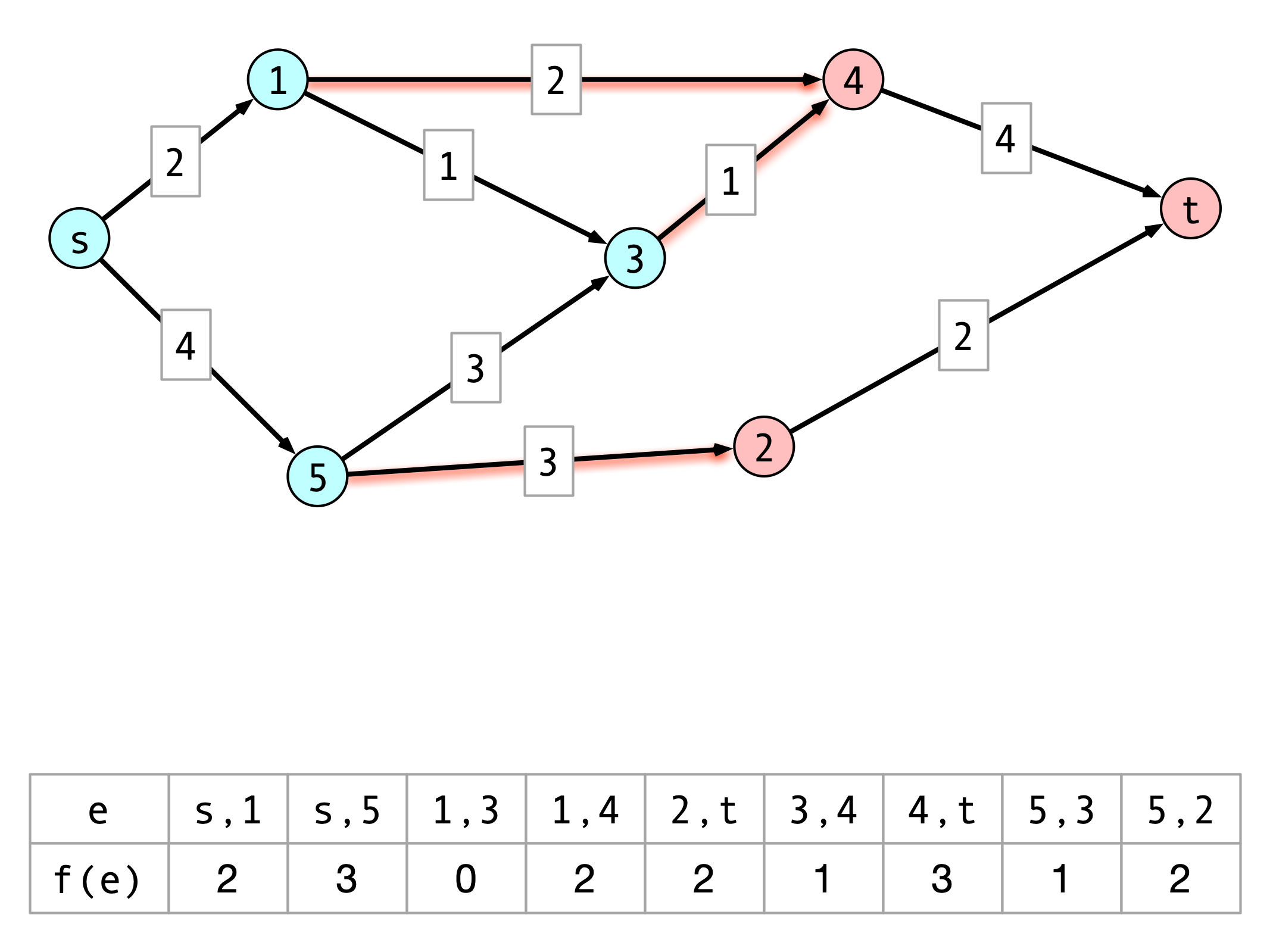## Correctness of Ford-Fulkerson

Idea. Relate values of flows to capacities of cuts:

• max flow = min cut

Outline:

• for any cut $(A, B)$, net flow across cut = value of flow
• $\implies$ max flow $\leq$ min cut
• if $f$ has no augmenting path in residual graph, then there is a cut with net flow = value of cut
• $\implies$ value of $f$ = capacity of cut
• $\implies \val(f) = \capacity(A, B) \geq$ min cut

Together these imply Ford-Fulkerson produces max flow

## Max Flow/Min Cut Example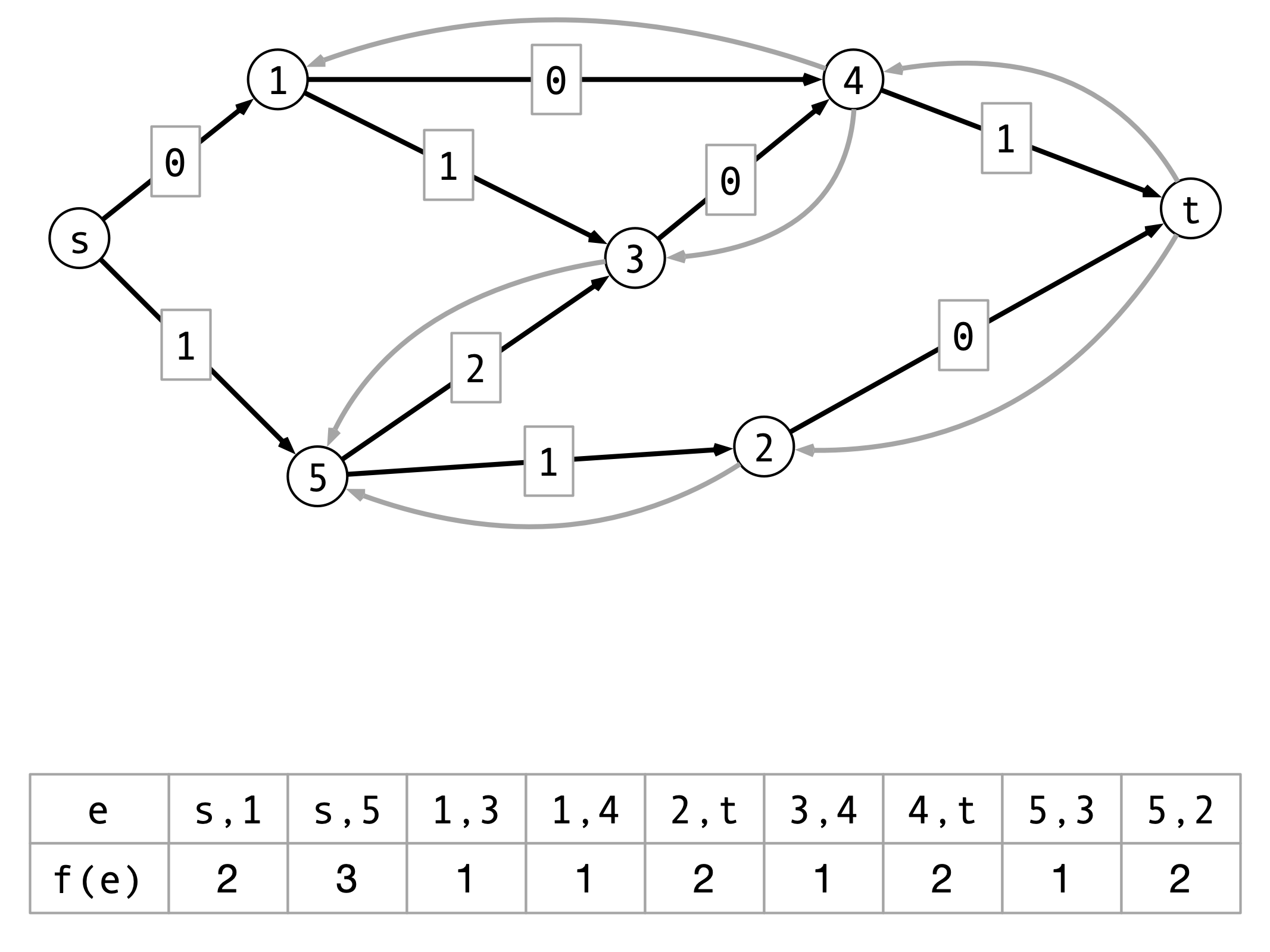## Claim 1

For any $s$ - $t$ cut $(A, B)$ and flow $f$, $\val(f) = f^{\text{out}}(A) - f^{\text{in}}(A)$

• $f^{\text{out}}(A) =$ flow out of $A$
• $f^{\text{in}}(A) =$ flow into $A$

Consequence. For all cuts $(A, B)$, $\val(f) \leq \capacity(A, B)$

## Claim 1 Illustration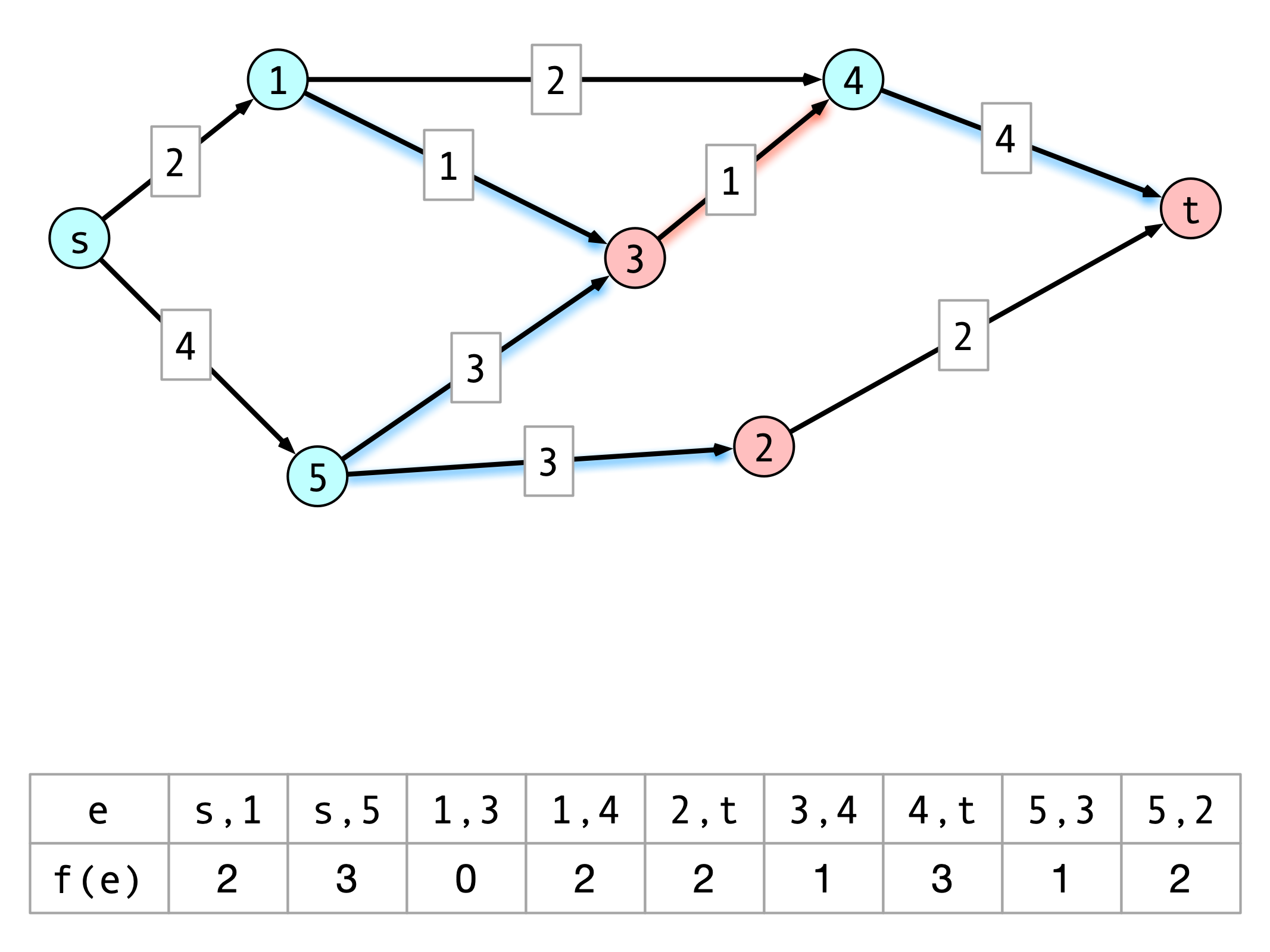## Claim 2

Suppose $f$ does not have an augmenting path in the auxiliary graph.

• $A^* =$ nodes reachable from $s$ in auxiliary graph
• $B^* =$ nodes not reachable

Then $\val(f) = \capacity(A^*, B^\star)$

## Claim 2 Illustration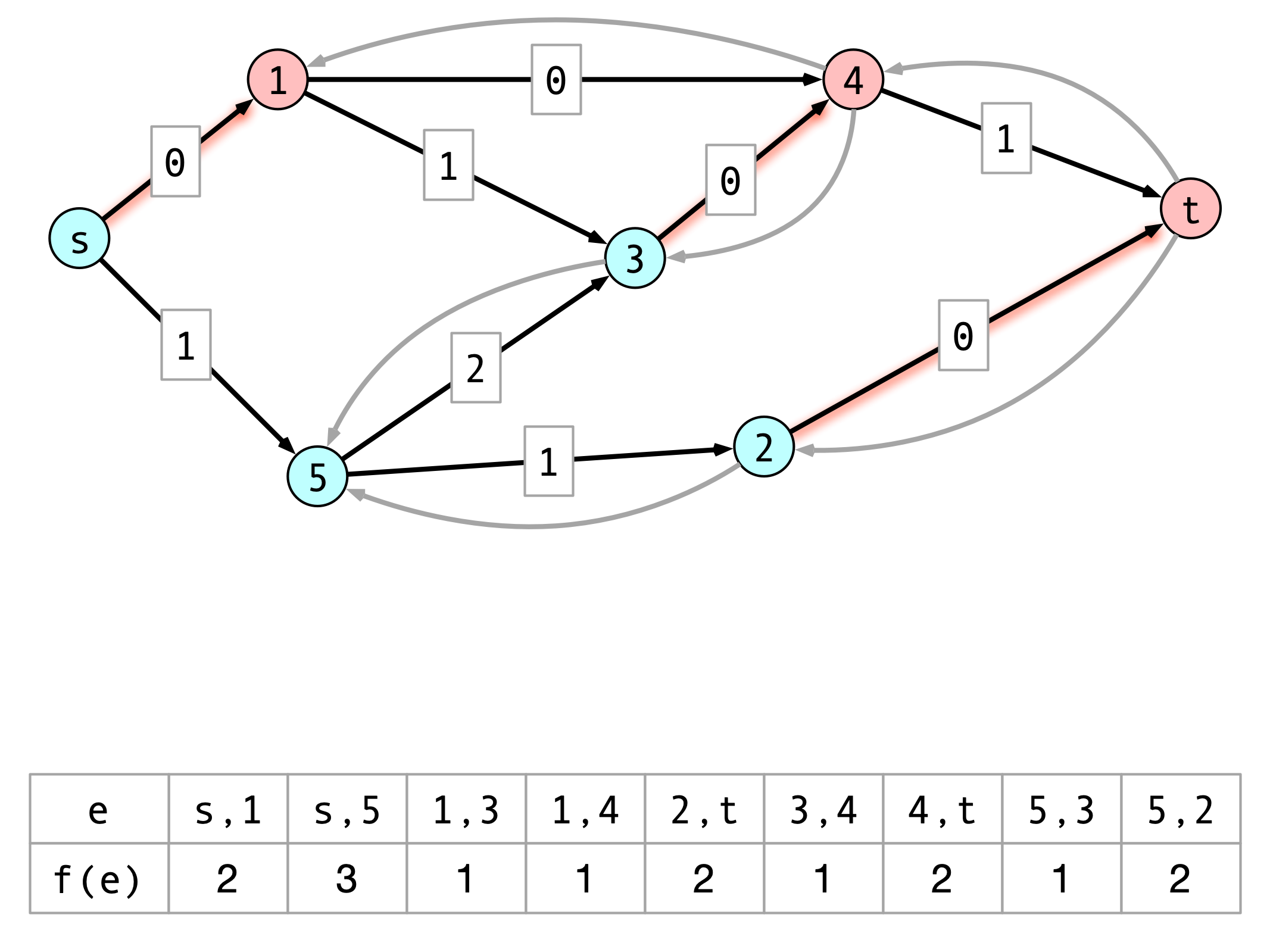## Correctness Follows

Consider flow $f$ found by Ford-Fulkerson.

1. By claim 1, no flow can have value larger than any cut capacity
2. By claim 2, $\val(f) = \capacity(A, B)$

These imply:

1. $f$ is a maximum flow
2. $(A, B)$ is a minimum cut

## Conclusion

$G = (V, E)$ a weighted, directed graph with minimum cut capacity $C$.

Ford-Fulkerson finds maximum flow in time $O(C m)$.

• can be modified to find minimum cut as well

## Next Time

1. Midterm on Wednesday
2. Stable Matching + Will’s research on Friday
3. Reductions and NP completeness after break